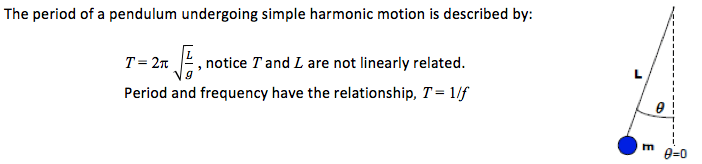This activity is not yet in ASA lesson plan formatting.

Students investigate a spring, a pendulum and pasta w/raisins or marshmallows to determine if the period depends on length, amplitude and mass for each oscillator. This can be used as the second half after the “Spring to Another World” lesson.

#### Simple Harmonic Motion

Simple harmonic motion (SHM) is the motion of an object subject to a force that is proportional to the object’s displacement. One example of SHM is the motion of a mass attached to a spring. In this case, the relationship between the spring force and the displacement is given by Hooke’s Law, F = -kx, where k is the spring constant, x is the displacement from the equilibrium length of the spring, and the minus sign indicates that the force opposes the displacement. In this laboratory you will study SHM as it applies to a:

1. Mass Attached to a Spring, and
2. Simple Pendulum

#### Mass Attached to a Spring

The motion of a mass attached to a spring is simple harmonic motion if:

1. there is no friction and
2. if the displacement of the mass from its equilibrium position at x = 0 is “small”. The displacement must be small enough so that the spring is not stretched beyond its elastic limit and becomes distorted.#### Procedure

Materials and equipment: big spring, weight hanger, masses, motion detector, and Logger Pro interface and software.

### A) Does the period of the motion depend on the amplitude?

STEP 1:

1. Please write down a prediction with a reason for question A.
2. Suspend the spring from the support rod.
3. From the lose end of the spring hang a 50g weight hanger with a 50g mass for a total of 100g.
4. Measure the position of the end of the spring relative to the floor or table top. This will be your spring/mass equilibrium position.
5. Pull the mass below the spring/mass equilibrium position.
6. Release the mass and use the motion detector to record the motion.
7. Use the motion data to determine the period of the motion. Describe how you used this data to determine the period.
8. How is the amplitude defined for this motion?
9. Repeat this procedure for five additional amplitudes. Record the amplitudes and the periods in a table.
10. Does your data indicate that the period of motion depends on the amplitude? Support your answer.
11. Theoretically (according to the equations), does the period of the motion depend on the amplitude? Support your answer.

STEP 2:

1. Please write down a prediction with a reason for question B
2. Experimentally determine the periods of motion for six masses 50g, 100g, 150g, 200g, 250g and 300g. Record the mass and the corresponding period in a table.
If you plot the non-linear function y = ax2 + b on a regular x, y graph, you would get a parabola. However, some forms of non-linear functions that are common in physics can be represented as straight lines. For example, if you plot y on the y-axis and x2 on the x-axis (instead of x), you would obtain a straight line.STEP 3: Using the equation relating period, mass and the spring constant, plot T2 on the vertical axis and m on the horizontal axis. What are the slope and y-intercept of the resulting line?

STEP 4:  Now generate the graph, and use its slope to determine k.

Simple Pendulum

The motion of a pendulum can be treated as simple harmonic if:

1. there is no friction and
2. if the displacement of the mass from the equilibrium position is small, q ≤ 15o#### Procedure

Materials and equipment: masses, string, stopwatch or other type of timer.

A) Finding the acceleration due to gravity, g

1. Attach one end of a string to the hook and hang a mass at the other end near the floor.
2. Taking note of the initial amplitude (remember for SHM motion q ≤ 15o), pull the mass to one side, release it, and determine the period of its motion.
3. Experimentally determine the periods of five simple pendulums, each with a different length, but the same mass. Use the distance from the point of support to the center of the mass as the length.  Record the lengths and the corresponding periods in a table.
4. Plot the data to yield a straight line, and then use the slope to determine g.
5. Use the percent error to compare your experimentally determined value of g to the accepted value.

B) Does the period of the motion depend on the mass?

1. Please write down a prediction with a reason for question D
2. Use the PhET simulation Pendulum Lab to investigate this question. Write a description of the procedure you followed and the data you collected to answer this question.

C) Does the period of motion depend on the Length?

1. Please write down a prediction with a reason for question E.
2. Using Pendulum Lab investigate this question. Write a description of the procedure you followed and the data you collected to answer this question.

#### Pasta and Raisins/Marshmallows

Place a raisin or marshmallow on the end of a stick of spaghetti. Shake your hand back and forth to make the pasta/raisin system oscillate.

A) Does the period depend on the mass?

B) Does the period depend on the length?

1. Do you think this system’s motion would fall under the classification of simple harmonic motion? Provide as much evidence as you can for your answer.
2. Do your answers to A and B above match a spring/mass system or a pendulum? How so?
3. Do you think this system can be modeled as a pendulum, spring, or neither? What are your reasons for each?